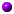Lecture notes for Math 259: Introduction to Analytic Number Theory (Spring 1998)

If you find a mistake, omission, etc., please let me know by e-mail.

The orange ball marks our current location in the course.

For an explanation of the background pattern, skip ahead to the end of the page.plan.ps and intro.ps: administrivia and philosophy/exampleselem.ps: Elementary methods I: variations on Euclideuler.ps: Elementary methods II: The Euler product for s>1 and consequencesdirichlet.ps: Dirichlet characters and L-series; Dirichlet's theorem modulo the non-vanishing of L-series at s=1chebi.ps: Cebysev's method; introduction of Stirling's approximation, and of the von Mangoldt function \Lambda(n) and its sum \psi(x)click here For Erdos' simplification of Cebysev's proof of the "Bertrand Postulate": there exists a prime between x and 2x for all x>1. Adapted from Hardy and Wright, pages 343-344.psi.ps: Complex analysis enters the picture via the contour integral formula for \psi(x) and similar sumszeta1.ps: The functional equation for the Riemann zeta function using Poisson inversion on theta series; basic facts about \Gamma(s) as a function of a complex variable s [corrected March 5]gamma.ps: The infinite product and asymptotic series for \Gamma(s) and some consequences [corrected Feb.23]prod.ps: Functions of finite order: Hadamard's product formula and its logarithmic derivativezeta2.ps: The Hadamard products for \xi(s) and \zeta(s); vertical distribution of the zeros of \zeta(s).free.ps: The nonvanishing of \zeta(s) on the edge \sigma=1 of the critical strip, and the classical zero-free region 1-\sigma << 1/log|t| for \zeta(s)pnt.ps: Conclusion of the proof of the Prime Number Theorem with error bound; some consequences and equivalents of the Riemann Hypothesis.Here's a bibliography of fast computations of \pi(x).lsx.ps: L(s,\chi) as an entire function [\chi a nontrivial primitive character mod q]; Gauss sums, and the functional equation relating L(s,\chi) with L(1-s,\bar\chi) [corrected March 9]pnt_q.ps: Product formula for L(s,\chi), and ensuing partial-fraction decomposition of its logarithmic derivative; a (bad!) zero-free region for L(s,\chi), and resulting estimates on \psi(x,\chi) and thus on \psi(x; a mod q) and \pi(x; a mod q). The Extended Riemann Hypothesis and consequences.Here's the PostScript source for "Chebyshev's Bias" by M.Rubinstein and P.Sarnak.free_q.ps: The classical region 1-\sigma << 1/log(q|t|+2) free of zeros of L(s,\chi) with at most one exception \beta; the resulting asymptotics for \psi(x; a mod q) etc.; lower bounds on 1-\beta and L(1,\chi), culminating with Siegel's theorem.l1x.ps: Closed formulas for L(1,chi) and their relationship with cyclotomic units, class numbers, and the distribution of quadratic residues.weyl.ps: Introduction to exponential sums; Weyl's equidistribution theoremkmv.ps: Kuzmin's inequality on sum(e(c_n)) with c_n in a nearly arithmetic progression; the Montgomery-Vaughan inequality on the mean square of an exponential sumvdc.ps: The van der Corput estimates and some applicationsmany_pts.ps: How many points can a curve of genus g have over the finite field of q elements? The zeta function of a curve over a finite field; the Weil and Drinfeld-Vladut bounds, and related matters.disc.ps: Stark's analytic lower bound on the absolute value of the discriminant of a number field (assuming GRH).Here are some tables of number fields, compiled by Henri Cohen.kloos.ps: An application of Weil's bound on Kloosterman sums, which we'll use again to estimate coefficients of modular forms.Here are the PostScript figures distributed in class with plots of
xy = c mod p for p=691 and 5077.[Introduction to modular forms for the full modular group PSL_2(Z), from Serre's A Course in Arithmetic, Chapter VII.]

For elementary proofs of the identities between Eisenstein series, see
Skoruppa, N.P.: A quick combinatorial proof of Eisenstein series idenities, J. Number Theory 43 (1993), 68-73
and references contained therein. [Robin Chapman (rjc@maths.exeter.ac.uk) recently posted this reference to sci.math.research.]poincare.ps: Proof of the bound O(n^(k-1/4+epsilon)) on the coefficients of a PSL_2(Z) cusp form of weight 2k, using Poincare series and bounds on Kloosterman sumssieve.ps: The Selberg (a.k.a. quadratic) sieve and some applications